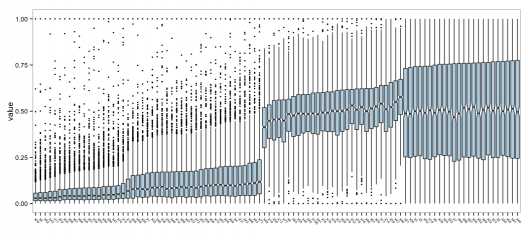Want to share your content on R-bloggers? click here if you have a blog, or here if you don't.

recent post to the SAS blog on
An exploratory technique for visualizing the distributions of 100
variables
. It’s a very succinct tutorial on both the power of
boxplots and how to make them in SAS (of course). I’m not one to let R
be “out-boxed”, so I threw together a quick re-creation of his example,
mostly as tutorial for any nascent R folks that come across it. (As an
aside, I catch Rick’s and other cool, non-R stuff via the Stats
Blogs
blog aggregator.)

The R implementation (syntax notwithstanding) is extremely similar.
First, we’ll need some packages to assist with data reshaping and pretty
plotting:

``` library(reshape2) library(ggplot2) ```

Then, we setup a list so we can pick from the same four distributions
and set the random seed to make this example reproducible:

``` dists <- c(rnorm, rexp, rlnorm, runif)   set.seed(1492) ```

Now, we generate a data frame of the `100` variables with `1,000`
observations, normalized from `0`-`1`:

``` many_vars <- data.frame(sapply(1:100, function(x) {   # generate 1,000 random samples tmp <- sample(dists, 1)[](1000)   # normalize them to be between 0 & 1 (tmp - min(tmp)) / (max(tmp) - min(tmp))   })) ```

The `sapply` iterates over the numbers `1` through `100`, passing each
number into a function. Each iteration samples an object from the
`dists` list (which are actual R functions) and then calls the function,
telling it to generate `1,000` samples and normalize the result to be
values between `0` & `1`. By default, R will generate column names that
begin with `X`:

``` str(many_vars[1:5]) # show the structure of the first 5 cols   ## 'data.frame': 1000 obs. of 5 variables: ## \$ X1: num 0.1768 0.4173 0.5111 0.0319 0.0644 ... ## \$ X2: num 0.217 0.275 0.596 0.785 0.825 ... ## \$ X3: num 0.458 0.637 0.115 0.468 0.469 ... ## \$ X4: num 0.5186 0.0358 0.5927 0.1138 0.1514 ... ## \$ X5: num 0.2855 0.0786 0.2193 0.433 0.9634 ... ```

We’re going to plot the boxplots, sorted by the third quantile (just
like in Rick’s example), so we’ll calculate their rank and then use
those ranks (shortly) to order a factor varible:

``` ranks <- names(sort(rank(sapply(colnames(many_vars), function(x) { as.numeric(quantile(many_vars[,x], 0.75)) })))) ```

There’s alot going on in there. We pass the column names from the
`many_vars` data frame to a function that will return the quantile we
want. Since `sapply` preserves the names we passed in as well as the
values, we extract them (via `names`) after we rank and sort the named
vector, giving us a character vector in the order we’ll need:

``` str(ranks)   ## chr [1:100] "X29" "X8" "X92" "X43" "X11" "X52" "X34" ... ```

Just like in the SAS post, we’ll need to reshape the data into long
format from wide
format
,
which we can do with `melt`:

``` many_vars_m <- melt(as.matrix(many_vars))   str(many_vars_m)   ## 'data.frame': 100000 obs. of 3 variables: ## \$ Var1 : int 1 2 3 4 5 6 7 8 9 10 ... ## \$ Var2 : Factor w/ 100 levels "X1","X2","X3",..: 1 1 1 1 1 1 1 1 1 1 ... ## \$ value: num 0.1768 0.4173 0.5111 0.0319 0.0644 ... ```

And, now we’ll use our ordered column names to ensure that our boxplots
will be presented in the right order (it would be in alpha order if
not). Factor variables in R are space-efficient and allow for handy
manipulations like this (amongst other things). By default,
`many_vars_m\$Var2` was in alpha order and this call just re-orders that
factor.

``` many_vars_m\$Var2 <- factor(many_vars_m\$Var2, ranks)   str(many_vars_m)   ## 'data.frame': 100000 obs. of 3 variables: ## \$ Var1 : int 1 2 3 4 5 6 7 8 9 10 ... ## \$ Var2 : Factor w/ 100 levels "X29","X8","X92",..: 24 24 24 24 24 24 24 24 24 24 ... ## \$ value: num 0.1768 0.4173 0.5111 0.0319 0.0644 ... ```

Lastly, we plot all our hard work (click/touch for larger version):

``` gg <- ggplot(many_vars_m, aes(x=Var2, y=value)) gg <- gg + geom_boxplot(fill="#BDD7E7", notch=TRUE, outlier.size=1) gg <- gg + labs(x="") gg <- gg + theme_bw() gg <- gg + theme(panel.grid=element_blank()) gg <- gg + theme(axis.text.x=element_text(angle=-45, hjust=0.001, size=5)) gg ```Here’s the program in it’s entirety:

``` library(reshape2) library(ggplot2)   dists <- c(rnorm, rexp, rlnorm, runif)   set.seed(1) many_vars <- data.frame(sapply(1:100, function(x) { tmp <- sample(dists, 1)[](1000) (tmp - min(tmp)) / (max(tmp) - min(tmp)) }))   ranks <- names(sort(rank(sapply(colnames(many_vars), function(x) { as.numeric(quantile(many_vars[,x], 0.75)) }))))   many_vars_m <- melt(as.matrix(many_vars))   many_vars_m\$Var2 <- factor(many_vars_m\$Var2, ranks)   gg <- ggplot(many_vars_m, aes(x=Var2, y=value)) gg <- gg + geom_boxplot(fill="steelblue", notch=TRUE, outlier.size=1) gg <- gg + labs(x="") gg <- gg + theme_bw() gg <- gg + theme(panel.grid=element_blank()) gg <- gg + theme(axis.text.x=element_text(angle=-45, hjust=0.001)) gg ```

I tweaked the boxplot, using a
notch
and making the outliers take up a fewer pixels.

I’m definitely in agreement with Rick that this is an excellent way to
compare many distributions.

Bonus points for the commenter who shows code to color the bars by which distribution generated them!